# Chapter 12: Introduction to probability theory - Online Test

Q1. Four persons are selected at random from a group of 3 men, 2 women and 4 children. The probability that exactly two of them are children is
Explaination / Solution:Q2. A number is selected from the set { 1, 2, 3,..., 20 }. The probability that the selected number is divisible by 3 or 4 is
Explaination / Solution: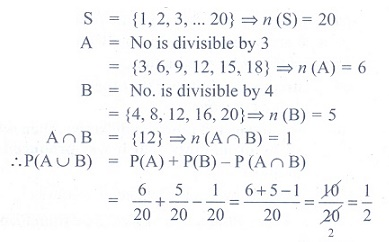Q3. A, B, and C try to hit a target simultaneously but independently. Their respective probabilities of hitting the target are 3/4 , 1/2 , 8/5 . The probability that the target is hit by A or B but not by C is
Explaination / Solution: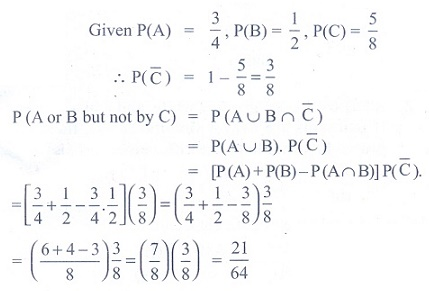Q4. If A and B are any two events, then the probability that exactly one of them occur is
Explaination / Solution: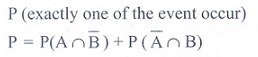Q5. Let A and B be two events such that P() = 1/6 , P ( A ∩ B) = 1/4 and P (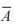) = 1/4 . Then the events A and B are
Explaination / Solution: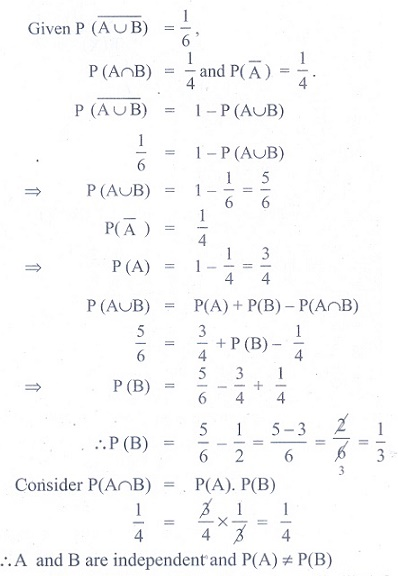Q6. Two items are chosen from a lot containing twelve items of which four are defective, then the probability that at least one of the item is defective
Explaination / Solution:Q7. A man has 3 fifty rupee notes, 4 hundred rupees notes and 6 five hundred rupees notes in his pocket. If 2 notes are taken at random, what are the odds in favour of both notes being of hundred rupee denomination?
Explaination / Solution: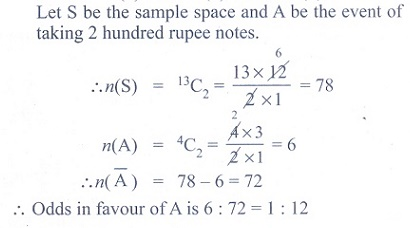Q8. A letter is taken at random from the letters of the word ‘ASSISTANT’ and another letter is taken at random from the letters of the word ‘STATISTICS’. The probability that the selected letters are the same is
Explaination / Solution: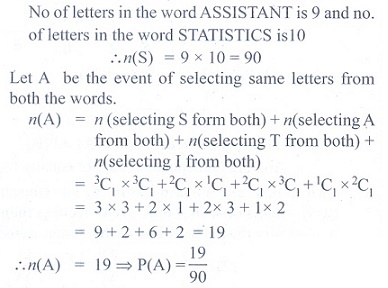Q9. A matrix is chosen at random from a set of all matrices of order 2, with elements 0 or 1 only. The probability that the determinant of the matrix chosen is non zero will be
Explaination / Solution: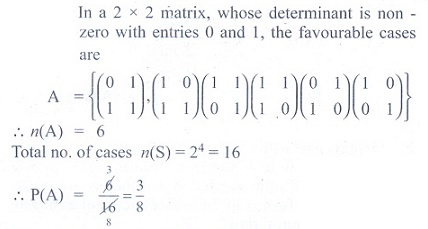Q10. A bag contains 5 white and 3 black balls. Five balls are drawn successively without replacement. The probability that they are alternately of different colours is
Explaination / Solution: